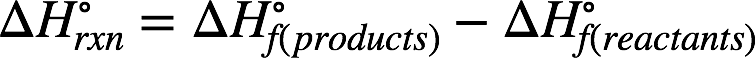# Problem: Some automobiles and buses have been equipped to burn propane (C3H8). Compare the amounts of energy that can be obtained per gram of C3H8(g) and per gram of gasoline, assuming that gasoline is pure octane, C8H18(l). (See Example 7‑11.) Look up the boiling point of propane. What disadvantages are there to using propane instead of gasoline as a fuel?

###### FREE Expert Solution

Recall: The enthalpy of reaction (ΔH˚rxn) can be calculated from the reactants' and products' enthalpy of formation (∆H˚f) using the equation:100% (126 ratings)###### Problem Details

Some automobiles and buses have been equipped to burn propane (C3H8). Compare the amounts of energy that can be obtained per gram of C3H8(g) and per gram of gasoline, assuming that gasoline is pure octane, C8H18(l). (See Example 7‑11.) Look up the boiling point of propane. What disadvantages are there to using propane instead of gasoline as a fuel?